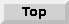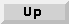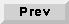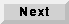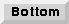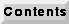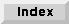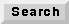17.2 An overview of C50 stars

The "open-palette" command in pigi ("O") will open a checkbox window that you can use to open the standard palettes in all of the installed domains. For the C50 domain, the star library is large enough that it has been divided into sub-palettes as was done with the SDF main palette.

The top-level palette is shown in figure 17-1. The palettes are Signal Sources, I/O, Arithmetic, Nonlinear Functions, Logic, Control, Conversion, Signal Processing, and Higher Order Functions. The stars on the Higher Order Functions (HOF) palette are used to help lay out schematics graphically. The HOF stars are in the HOF domain, and not the C50 domain. Each palette is summarized in more detail below. More information about each star can be obtained using the on-line "profile" command (","), the on-line "man" command ("M"), or by looking in the Star Atlas volume of The Almagest.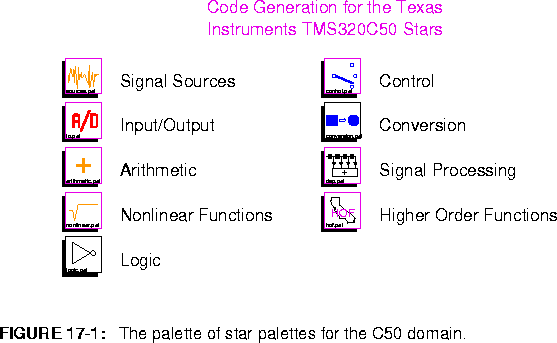BlackHole Discard all inputs. This star is useful for discarding signals that are not useful.

17.2.1 Source stars

Source stars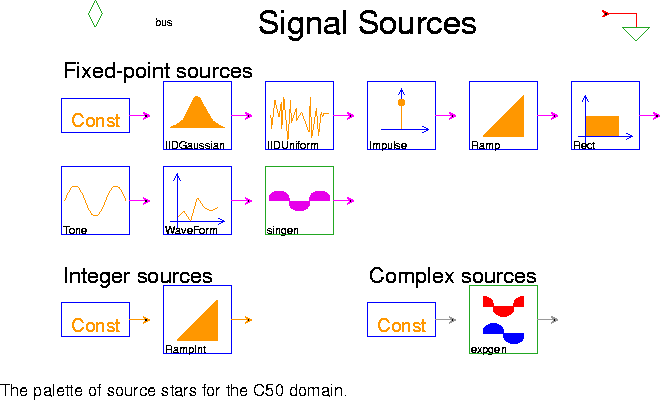are stars with only outputs. They generate signals, and may represent external inputs to the system, constant data, or synthesized stimuli. The palette of source stars is shown in figure
17-2. Refer to 5.2.1 on page 5-5 for descriptions of the SDF equivalent stars: Const, ConstCx, ConstInt, Ramp, RampInt, Rect, singen, and WaveForm.

Impulse Generate a single impulse or an impulse train. The size is determined by impulseSize (default ONE). If period (default is 0) is positive an impulse train with this period is generated, otherwise a single impulse is generated. If delay (default 0) is positive the impulse (or impulse train) is delayed by this amount.
IIDUniform Generate an i.i.d. uniformly distributed pseudo-random process. Output is uniformly distributed between -range and range (default ONE).
IIDGaussian Generate a white Gaussian pseudo-random process with mean 0 and standard deviation 0.1. A Gaussian distribution is realized by summing noUniforms (default 16) number of uniform random variables. According to the central limit theorem, the sum of N random variables approaches a Gaussian distribution as N approaches infinity.
Tone Generate a sine or cosine wave using a second order oscillator. The wave will be of amplitude (default 0.5), frequency (default 0.2), and calcType (default "sin")

17.2.2 I/O Stars

I/O stars are target specific stars that allow input and output of stimuli to a target architecture. Currently there are I/O stars only for the C50 DSK board so these stars should only be used with the DSKC50 target. These stars are located on the TI 320C5x IO palette inside the Input/Output palette.

AIn This is an interrupt driven star to receive samples from the A/D converter in the Analog Interface Chip. The sample rate is determined by sampleRate. The actual conversion rate is 285.7KHz/N where N is an integer from 4 to 64. This star supports an internal buffer to hold the received samples. The size of this buffer can be set manually by changing the interruptBufferSize parameter. Setting interruptBufferSize to a negative value will set the size of the buffer equal to the number of times the star is fired on each iteration of the universe.
AOut This is an interrupt driven star to send samples to the D/A converter in the AIC chip. The parameters are identical to those of the AIn star.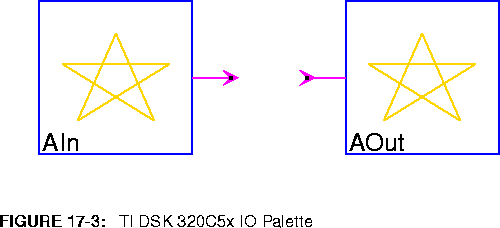17.2.3 Arithmetic stars

The arithmetic stars that are available are shown in figure 17-4.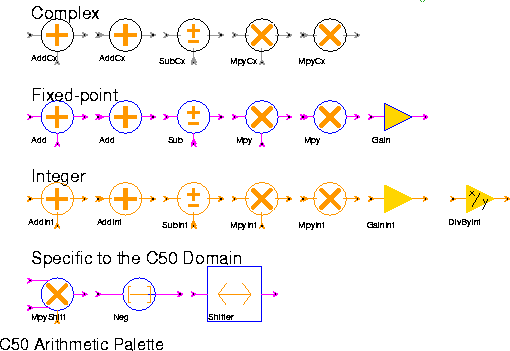Add (2 icons) Output the sum of the inputs. If saturation is set to yes, the output will saturate.
Sub Outputs the "pos" input minus all of the "neg" inputs.
Mpy (2 icons) Outputs the product of all of the inputs.
Gain The output is set the input multiplied by a gain term. The gain must be in [-1,1).
AddCx (2 icons) Output the complex sum of the inputs. If saturation is set to yes, the output will saturate.
SubCx Outputs the "pos" input minus all of the "neg" inputs.
MpyCx (2 icons) Outputs the product of all of the inputs.
AddInt (2 icons) Output the sum of the inputs. If saturation is set to yes, the output will saturate.
SubInt Outputs the "pos" input minus all of the "neg" inputs.
MpyInt (2 icons) Outputs the product of all of the inputs.
GainInt The output is set the input multiplied by an integer gain term.
DivByInt This is an amplifier. The integer output is the integer input divided by the integer divisor (default 2). Truncated integer division is used.
MpyShift Multiply and shift.
Neg Output the negation of the input.
Shifter Scale by shifting left leftShifts bits. Negative values of leftShifts implies right shifting.

17.2.4 Nonlinear stars

The nonlinear palette (figure 17-5) in the C50 domain includes transcendental functions, quantizers, table lookup stars, and miscellaneous nonlinear functions.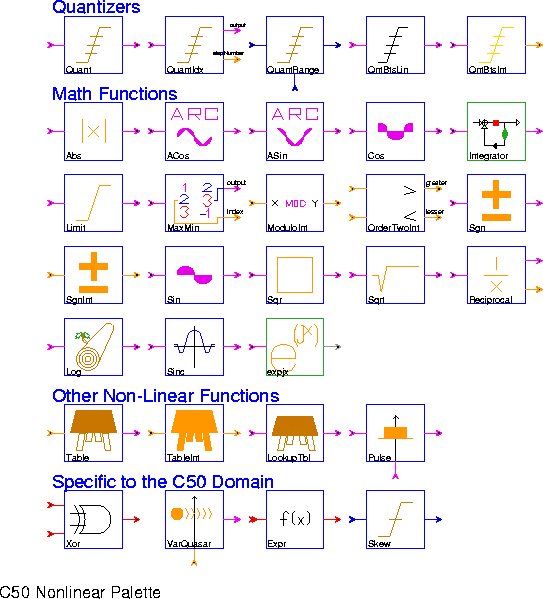Abs Output the absolute value of the input.
ACos Output the inverse cosine of the input, which is in the range -1.0 to 1.0. The output, in the principle range of 0 to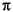, is scaled down by.
ASin Output the inverse sine of the input, which is in the range -1.0 to 1.0. The output, in the principle range of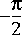to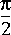, is scaled down by.
Cos Output the cosine, calculated the table lookup. The input range is [-1,1] scaled by.
expjx Output the complex exponential of the input.
Intgrtr An integrator with leakage set by feedbackGain. If there is an overflow, the onOverflow parameter will designate a wrap around, saturate or reset operation.
Limit Limits the input between the range of [bottom, top].
Log Outputs the base two logarithm.
MaxMin Output the maximal or minimal (MAX) sample out of the last N input samples. This can either compareMagnitude or take into account the sign. If outputMagnitude is YES the magnitude of the result is written to the output, otherwise the result itself is written.
ModuloInt Output the remainder after dividing the integer input by the integer modulo parameter.
OrderTwoInt Takes two inputs and outputs the greater and lesser of the two integers.
Quant Quantizes the input to one of N+1 possible output levels using N thresholds.
QuantIdx The star quantizes the input to one of N+1 possible output levels using N thresholds. It also outputs the index of the quantization level used.
QuantRange Quantizes the input to one of N+1 possible output levels using N thresholds.
Reciprocal Outputs the reciprocal to Nf precision in terms of a fraction and some left shifts.
Sgn Outputs the sign of the input.
SgnInt Outputs the sign of the integer input.
Sin Outputs the sine, calculated using a table lookup. The input range is [-1,1) scaled by.
Sinc Outputs the sinc functions calculated as sin(x)/x.
Sqrt Outputs the square root of the input.
Table Implements a real-valued lookup table. The values state contains the values to output; its first element is element zero. An error occurs if an out of bounds value is received.
TableInt Implements an integer-valued lookup table. The values state contains the values to output; its first element is element zero. An error occurs if an out of bounds value is received.
Expr General expression evaluation.
LookupTbl The input accesses a lookup table. The interpolation parameter determines the output for input values between table-entry points. If interpolation is "linear" the star will interpolate between table entries; if interpolation is set to "none", it will use the next lowest entry.
Pulse Generates a variable length pulse. A pulse begins when a non-zero trigger is received. The pulse duration varies between 1 and maxDuration as the control varies between [-1,1).
QntBtsInt Outputs the two's complement number given by the top noBits of the input (for integer output).
QntBtsLin Outputs the two's complement number given by the top noBits of the input, but an optional offset can be added to shift the output levels up or down.
Skew Generic skewing star.
Sqr Outputs the square of the input.
VarQuasar A sequence of values(data) is repeated at the output with period N (integer input), zero-padding or truncating the sequence to N if necessary. A value of O for N yields an aperiodic sequence.
Xor Output the bit-wise exclusive-or of the inputs.

17.2.5 Logic stars

The Logic stars are discussed below: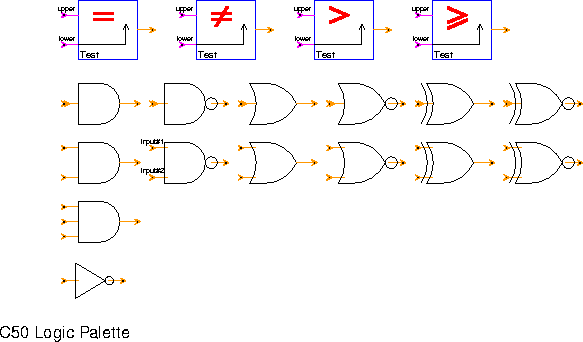Test (4 icons) Test to see if two inputs are equal, not equal, greater than, and greater than or equal. For less than and less than or equal, switch the order of the inputs.
And (3 icons) True if all inputs are non-zero.
Nand (2 icons) True if all inputs are not non-zero.
Or (2 icons) True if any input is non-zero.
Nor (2 icons) True if any input is zero.
Xor (2 icons) True if its inputs differ in value.
Xnor (2 icons) True if its inputs coincide in value.
Not Logical inverter.

17.2.6 Control stars

Control stars (figure 17-7)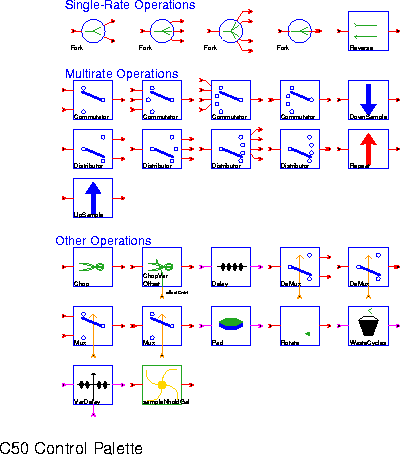manipulate the flow of tokens. All of these stars are polymorphic; they operate on any data type. Refer to 5.2.6 on page 5-17 for descriptions of the SDF equivalent stars: Fork, DownSample, Commutator, Distributor, Mux, Repeat, Reverse, and UpSample.

ChopVarOffset This star has the same functionality as the Chop star except now the offset parameter is determined at run time through a control input.
Cut On each execution, this star reads a block of nread samples (default 128) and writes nwrite of these samples (default 64), skipping the first offset samples (default 0). It is an error if nwrite + offset > nread. If nwrite > nread, then the output consists of overlapping windows, and hence offset must be negative.
Delay A delay star of parameter totalDelay unit delays.
Pad On each execution, Pad reads a block of nread samples and writes a block of nwrite samples. The first offset samples have value fill, the next nread output samples have values taken from the inputs, and the last nwrite - nread - offset samples have value fill again.
Rotate The star reads in an input block of a certain length and performs a circular shift of the input. If the rotation is positive, the input is shifted to the left so that ouput = input[rotation]. If the rotation is negative, the input is shifted to the right so that output[rotation] = input.
sampleNholdGalaxy
This sample-and-hold galaxy is more memory efficient than using a downsample star for the same purpose. This star is not present in Ptolemy0.6.
VarDelay A variable delay that will vary between 0 and maxDelay as the control input varies between -1.0 and 1.0.
WasteCycles Stalls the flow of data for cyclesToWaste number of cycles.

17.2.7 Conversion stars

The palette in figure 17-8 shows stars for format conversions from fixed point to complex fixed point.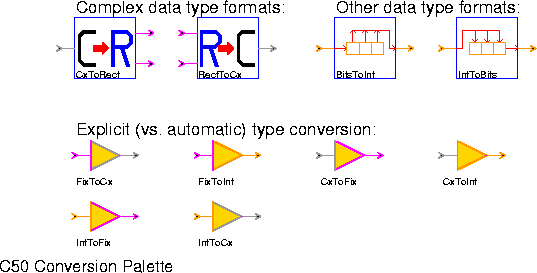CxToRect Output the real part and imaginary part of the input of separate output ports.
RectToCx Output a complex signal with real and imaginary part inputs.
BitsToInt Convert a stream of bits to an integer.
IntToBits Convert an integer into a stream of bits.
FixToCx Convert fixed-point numbers to complex fixed-point numbers.
FixToInt Convert fixed-point numbers to integer numbers.
CxToFix Output the magnitude squared of the complex number.
CxToInt Output the magnitude squared of the complex number.
IntToFix Convert an integer input to a fixed point output.
IntToCx Convert an integer input to a complex output.

17.2.8 Signal processing stars

The palette shown in figure 17-9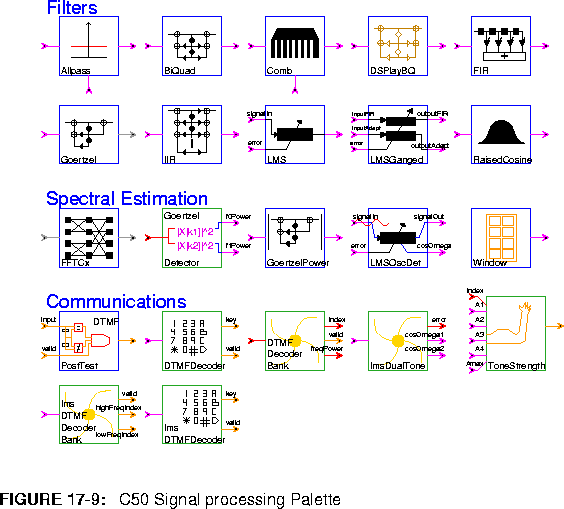has icons for the library of signal processing functions. The filter stars follow. The Goertzel and IIR stars are identical to their SDF counterparts.

Allpass An allpass filter with one pole and one zero. The location of these is given by the "polezero" input.Comb A comb filter with a one-pole lowpass filter in the delay loop.
BiquadDSPlay A two-pole, two zero IIR filter (a biquad). This biquad is tailored to use the coefficients from the DSPlay filter design tool. If DSPlay gives the coefficients: A B C D E then define the parameters as follows: a=A, b=B, c=C, d=-(D+1), e = -E. This only works if a, b, c, d, and e, are in the range [-1,1). The default coefficients implement a low pass filter.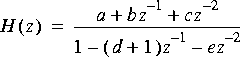FIR A finite impulse response (FIR) filter. Coefficients are specified by the taps parameter. The default coefficients give an 8th order, linear-phase, lowpass filter. To read coefficients from a file, replace the default coefficients with < filename, preferably specifying a complete path. Polyphase multirate filtering is not yet supported.
LMS An adaptive filter using the LMS adaptation algorithm. The initial coefficients are given by the coef parameter. The default initial coefficients give an 8th order, linear phase lowpass filter. To read default coefficients from a file, replace the default coefficients with < filename, preferably specifying a complete path. This star supports decimation, but not interpolation.
LMSGanged A LMS filter were the coefficients from the adaptive filter are used to run a FIR filter in parallel. The initial coefficients default to a lowpass filter of order 8.
RaisedCos An FIR filter with a magnitude frequency response shaped like the standard raised cosine used in digital communications.See the SDFRaisedCosine star for more information.
The spectral estimation stars follow. The GoertzelDetector, GoertzelPower, and LMSOscDet are identical to their SDF counterparts.

FFTCx Compute the discrete-time Fourier transform of a complex input using the fast Fourier transform (FFT) algorithm. The parameter order (default 8) is the transform size. The parameter direction (default 1) is 1 for forward, -1 for the inverse FFT.
Window Generate standard window functions or periodic repetitions of standard window functions. The possible functions are Rectangle, Bartlett, Hanning, Hamming, Blackman, SteepBlackman, and Kaiser. One period of samples is produced on each firing.
The communications stars are exactly like their SDF counterparts.